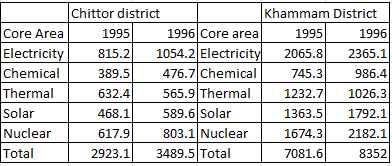### CAT 1996 Question Paper Question 65

Instructions

Answer the questions based on the following information. The amount of money invested (rupees in crores) in the core infrastructure areas of two districts, Chittoor and Khammam, Andhra Pradesh, is as follows.Question 65

# If the total investment in Khammam shows the same rate of increase in 1997, as it had shown from 1995 to 1996, what approximately would be the total investment in Khammam in 1997?

Solution

Increase in 1996 compared to 1995 for khammam = 8352 - 7081.6 = 1270.4
With approximation method, we can say that %increase is above 17 and lower than 18.
Hence for 1997 investment will be have an increment of 18% nearly
So in 1997, investment = $$8352 (1+ \frac{18}{100}) = 9855$$ crore (nearly)

• All Quant CAT Formulas and shortcuts PDF
• 30+ CAT previous papers with solutions PDF